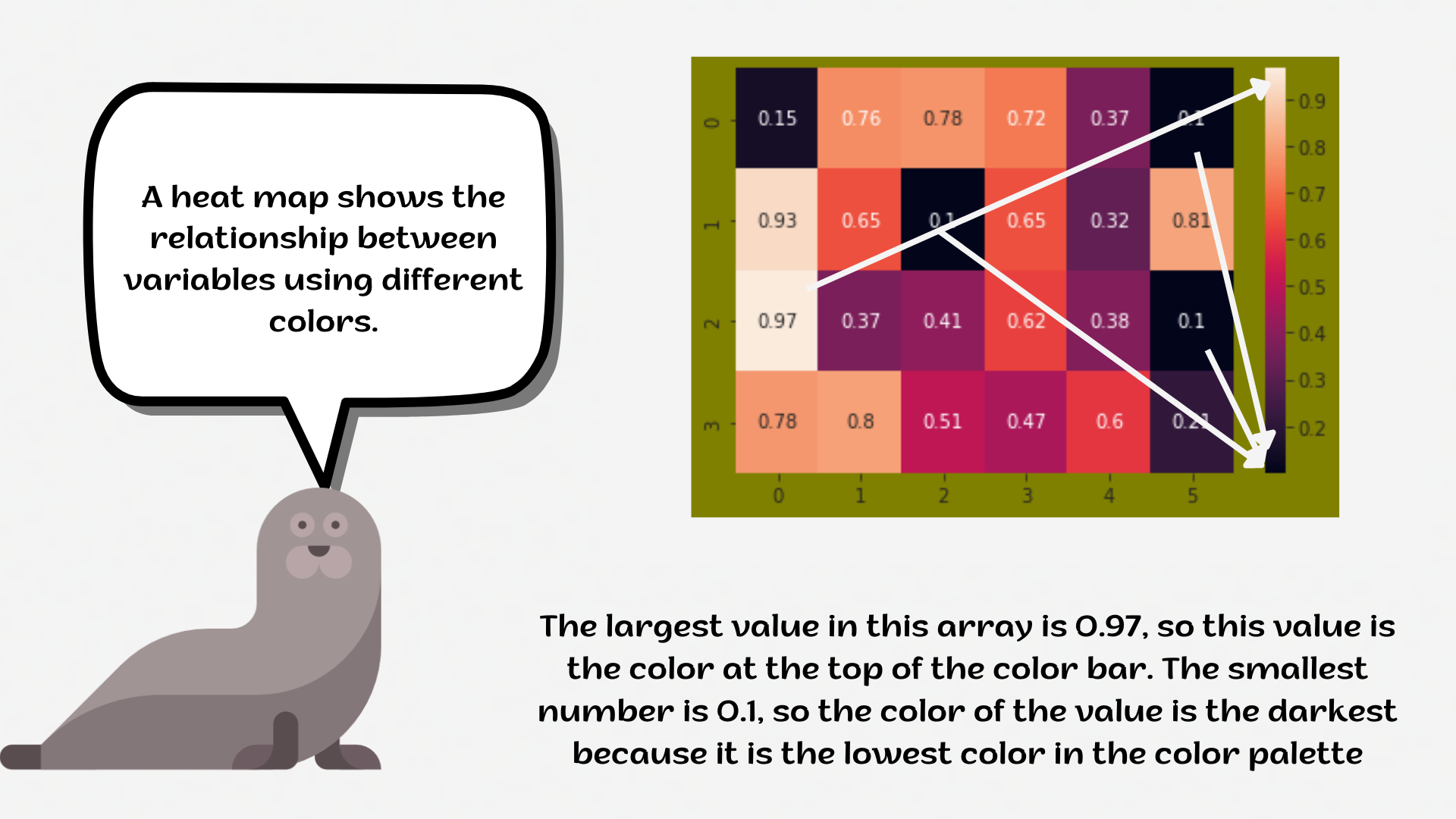Course Content

# Deep Dive into the seaborn Visualization

1. Light Start

2. Distributions of Data

3. Categorical Plot Types

4. Matrix Plots

5. Multi-Plot Grids

6. Regression Models

Deep Dive into the seaborn Visualization

##HeatmapA `heatmap` is a plot of rectangular data as a color-encoded matrix. As a parameter, it takes a 2D dataset. That dataset can be coerced into an `ndarray`.

This is a great way to visualize data because it can show the relation between variables, including time. For instance, the number of flights through the years.1. Set the `'ticks'` style with the `'seagreen'` `figure.facecolor`.
2. Create the `heatmap` using the `seaborn` library:
• Add the data for the `heatmap`. You only need to input the name of the DataFrame (without `data = ...`);
• Set the `'viridis'` `cmap` parameter;
• Add the `annot` parameter;
• Set the `fmt` parameter equals the `'0.99g'`;
• Set the `linecolor` parameter equals the `'plum'`;
• Display the plot.

Everything was clear?

Section 4. Chapter 1